# Predicting Vehicle Price With Random Forest Regressor

Predicting Selling Price | Regression Models | Cross ValidationSource: https://www.vwstaug.com/blogs/1984/best-practices-on-buying-a-car/how-to-choose-the-right-car-dealership/attachment/15075074577_bda5ec36c4_b/

In machine learning, there are classification and regression model. The different of the two is that classification predict the output (or y) as either yes or no, up or down, or some other categories. For example, in sentiment analysis, we want to know whether a review belongs to a good or a bad sentiment. However, in regression, the output that we want is the value, like what will be the price of the house.

In this vehicle price prediction, it is a good practice for the regression model. It contains several features where we need to prepare and we are also facing none normal distribution.

The aim of this article is to go through steps for doing regression analysis where we will compare several models and run them with cross-validation. So, stay tuned. Below is the list of what we will be covering.

# 1. Data Overview

Kaggle has provided a data set for second hand vehicles’ prices. We are going to use this data for our project. The full data set contains 423,857 rows of data with 25 attributes. Let’s have a look at it.

`import pandas as pdimport matplotlib.pyplot as pltimport seaborn as snsimport numpy as npdata = pd.read_csv('.../vehicles.csv')pd.set_option('display.max_columns', 25)data.info()`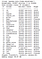Data Information

We can see that our data contain many missing values and mostly are object type of the categorial data. We are going to have a lot to do with data preparation. But before we go to that, we shall visualize our data.

# 2. Data Visualization

As we have longitude and latitude attributes, we can create geographically view from it.

`data.plot(kind=’scatter’, x=’long’, y=’lat’, alpha=0.4, figsize=(10,7))`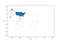Visualizing data with scatter plot

Our data is collected mostly within the USA. We can see this from the geographical visualisation as majority of the data are located in the USA area, and only few of them are scattered away from the main group.

How about how much attributes are correlated to each other?

`matrix = np.triu(data.corr())fig, ax = plt.subplots(figsize=(15,10))sns.heatmap(X_train.corr(), mask=matrix, ax=ax, cbar=True, annot=True, square=True)plt.savefig('heatmap.png')`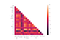Heatmap showing attributes correlation

# 3. Data Preparation

Firstly, we shall look at the relationship of the selling price with the year of the vehicles.

`from scipy import statsfrom scipy.stats import norm, skewfig, ax = plt.subplots()ax.scatter(x = data['year'], y = data['price'])plt.ylabel('SalePrice', fontsize=13)plt.xlabel('Year', fontsize=13)plt.show()`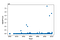Car selling price from each year

Seem that we are having outliers. To do that, we choose to delete data that are lower than the 1st quantile and higher than the 3rd quantile. After the deletion, we have 324,723 rows of data remained.

Then, we want to look at the distribution of the price. We can plot this by using the seaborn.

`sns.distplot(data['price'], fit=norm)fig = plt.figure()res = stats.probplot(data['price'], plot=plt)`

From this, we can see that data does not have normal distribution, it contains peakedness (having a peak), have positive skewness (tail on the right side is longer), and not on the diagonal line.

To solve this problem, we can apply log function to the price.

`data['price'] = np.log(data['price'])sns.distplot(data['price'], fit=norm)fig = plt.figure()res = stats.probplot(data['price'], plot=plt)`

Now, it is time to handling other attributes. But before we get into that, we will add one more column and call it ‘age’, and drop the year column. With this, we will get the age of the car. We do this as we want to know how old are those second hand cars.

`data['age'] = 2021 - data['year']`

## Data Cleaning Step 1

Then, with the data, we need to create train and test set. We do this with the train_test_split from sklearn.model_selection

After splitting the data, the technique that we are going to use to fill the missing value of the data are
1. fill the missing value with “None”,
2. fill the missing value with 0.0,
3. fill the missing value with mode(),
4. fill the missing value with most occurred categories, and
5. replace ‘yes’ for rows with values and ‘no’ for rows without values.

For filling the missing value with “None”, we apply them to ‘manufacturer’, ‘model’, ‘condition’, ‘title_status’, ‘drive’, ‘size’, ‘type’ and ‘paint_color’.

For filling the missing value with 0.0, we apply them to ‘odometer’, ‘lat’, ‘long’ and ‘age’.

For filling the missing value with mode(), we apply them to ‘cylinders’, ‘title_status’, ‘transmission’ and ‘fuel’.

For ‘cylinders’ attributes, we filled the none value with ‘other’.

For ‘fuel’ attributes, we filled the non value with ‘gas’.

For ‘transmission’, we filled the value with ‘automatic’.

For ‘vin’, we use re to find rows with value, where we replace the value with ‘yes’, and we filled non value rows with ‘no’.

These cleaning needs to be apply to both X_train and X_test set.

`import refrom sklearn.model_selection import train_test_splittrain, test = train_test_split(data)#Split the data setX_train = train.drop(['price'], axis='columns')y_train = train['price']X_test = test.drop(['price'], axis='columns')y_test = test['price']#Fill the missing value with “None”cols_for_none = ('manufacturer','model','condition','title_status','drive','size',                'type','paint_color')for c in cols_for_none:    X_train[c] = X_train[c].fillna("None")     X_test[c] = X_test[c].fillna("None") #Fill the missing value with 0.0cols_for_zero = ('odometer','lat','long','age')for c in cols_for_zero:    X_train[c] = X_train[c].fillna(0.0)    X_test[c] = X_test[c].fillna(0.0)   #Fill the missing value with mode()cols_for_mode = ('cylinders','title_status','transmission','fuel')for c in cols_for_mode:    X_train[c] = X_train[c].fillna(X_train[c].mode())    X_test[c] = X_test[c].fillna(X_test[c].mode())#Fill the missing value with most occurred categoriesX_train['cylinders'] = X_train['cylinders'].fillna("other")X_train['fuel'] = X_train['fuel'].fillna('gas')X_train['transmission'] = X_train['transmission'].fillna('automatic')X_test['cylinders'] = X_test['cylinders'].fillna("other")X_test['fuel'] = X_test['fuel'].fillna('gas')X_test['transmission'] = X_test['transmission'].fillna('automatic')#Replace ‘yes’ for rows with values and ‘no’ for rows without valuesX_train = X_train.replace({'vin':r'(\w*\S)'}, {'vin':"Yes"}, regex=True)X_train['vin'] = X_train['vin'].fillna("No")X_test = X_test.replace({'vin':r'(\w*\S)'}, {'vin':"Yes"}, regex=True)X_test['vin'] = X_test['vin'].fillna("No")`

## Data Cleaning Step 2

Now, we filled all the missing data, but before we can train our model, we need to turn all categories data into numerical values. We can do this by using LabelEncoder from sklearn.preprocessing. Categories that we need to manage are ‘region’, ‘manufacturer’, ‘model’, ‘condition’, ‘fuel’, ‘title_status’, ‘title_status’, ‘transmission’, ‘vin’, ‘drive’, ‘size’, ‘type’, ‘paint_color’, ‘state’ and ‘cylinders’.

For ‘odometer’, it also does not have normal distribution, therefore we need to turn them into normal distribution as well.

`from sklearn.preprocessing import LabelEncoderle = LabelEncoder()#Listing all the categorial attributescols = ['region','manufacturer','model','condition','fuel','title_status','title_status','transmission','vin',       'drive','size','type','paint_color','state','cylinders']#Apply to both X_train and X_test datafor c in cols:    le.fit(list(X_train[c].values))    X_train[c] = le.transform(list(X_train[c].values))    for c in cols:    le.fit(list(X_test[c].values))    X_test[c] = le.transform(list(X_test[c].values))#Manage the distribution type of odometer datalog_value = ('odometer')for c in log_value:    X_train[c] = np.log1p(X_train[c])    X_test[c] = np.log1p(X_test[c])`

Now, as all of our attributes are in numbers, we can look at how important each attribute is.

`from sklearn.feature_selection import SelectKBestfrom sklearn.feature_selection import f_regression#Show the important attributes in descending orderbest_features = SelectKBest(score_func=f_regression, k=18)top_features = best_features.fit(X_train,y_train)scores = pd.DataFrame(top_features.scores_)columns = pd.DataFrame(X_train.columns)featureScores = pd.concat([columns, scores], axis=1)featureScores.columns = ['Features','Scores']print(featureScores.nlargest(18, 'Scores'))`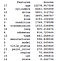Features important

# 4. Model Comparison and Evaluation

Most of the work have been done. Now, we can run several regression models to compare each model accuracy. With each model, we apply 5 folds cross-validation.

With cross-validate, our 5 K-folds, it randomly splits data into 5 folds and train the model 5 times. Every fold, will be picking for evaluation at each of different time while the other 4 will be kept for training.

Let’s understand the model that we are going to select.

## Random Forest Regressor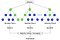Source: https://www.analyticsvidhya.com/blog/2020/05/decision-tree-vs-random-forest-algorithm/

This model trains multiple decision trees and the final result is the voting majority (for classification) or average voting (for regression). Each tree will draw random sample so this prevent overfitting and with large dataset, random forest seems to perform well.

## Gradient Boosting Regressor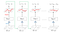Source: https://www.geeksforgeeks.org/ml-gradient-boosting/

The model prediction comes from the learning of the weak prediction from previous models. 1st tree prediction error r1 will be trained in the 2nd tree where it will get error r2. Then r2 is used to train in 3rd tree, and so on until it reach N trees.

## Linear Regression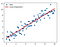Source: https://www.researchgate.net/figure/Linear-Regression-model-sample-illustration_fig3_333457161

The most commonly seen regression model of all. The prediction (y) is estimated from the independent variables (X1, X2, X3, … , Xn). The error is the distance of y from the linear regression line.

## Extreme Gradient Boosting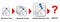Source: https://www.abiqos.com/2019/08/expand-your-predictive-palette-xgboost-in-alteryx/

Extreme Gradient Boosting (XGB) uses gradient boosting decision trees algorithms where its main objectives are the execution speed and the model performance. In recent years, many kaggle competition use XGB for their model.

Now, we lets look at the implementation and evaluation of each model.

`from sklearn.linear_model import LinearRegressionfrom sklearn.ensemble import RandomForestRegressor, GradientBoostingRegressorimport xgboost as xgbfrom sklearn.model_selection import cross_val_scorelr = LinearRegression()rr = RandomForestRegressor()gbr = GradientBoostingRegressor()xgb = xgb.XGBRegressor()#Create function to displaying scoresdef display_scores(scores):    print("Scores: ", scores)    print("Mean: ", scores.mean())    print("Standard Deviation: ", scores.std())#Training the Random Forest Regressorprint("Random Forest Regressor Scores")scores = cross_val_score(rr, X_train, y_train, scoring='neg_mean_squared_error', cv=5)random_forest_scores = np.sqrt(-scores)display_scores(random_forest_scores)print("\n")#Training the Gradient Boosting Regressorprint('Gradient Boosting Regressor Scores')scores = cross_val_score(gbr, X_train, y_train, scoring='neg_mean_squared_error', cv=5)gradient_boosting_regressor = np.sqrt(-scores)display_scores(gradient_boosting_regressor)print("\n")#Training the Linear Regressionprint('Linear Regression Scores')scores = cross_val_score(lr, X_train, y_train, scoring='neg_mean_squared_error', cv=5)linear_regression = np.sqrt(-scores)display_scores(linear_regression)print("\n")#Training the Extreme Gradient Boostingprint("xGB Scores")scores = cross_val_score(xgb, X_train, y_train, scoring='neg_mean_squared_error', cv=5)xgb_regressor = np.sqrt(-scores)display_scores(xgb_regressor)`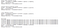Scores from different regression models.

From this, we can see that our random forest regressor receives the best score with mean of 0.3822 and standard deviation of 0.0027. Next best performer is the Gradient Boosting Regressor with the mean of 0.4471 and standard deviation of 0.0028. Third winner is the XGB with mean of 0.4473 and standard deviation of 0.0023.

# 5. Prediction

From our training model, Random Forest Regressor has the best performance with the lower mean error. With this, we will used them for the prediction.

`#Do the predictionrr.fit(X_train, y_train)pred = rr.predict(X_test)#Convert the log value back to the original valuey_test = np.exp(y_test)pred = np.exp(pred)#Calculate the error and accuracyerrors = abs(pred - y_test)print('Average absolute error:', round(np.mean(errors), 2), 'degrees.')mape = 100*(errors / y_test)accuracy = 100 - np.mean(mape)print('Accuracy:', round(accuracy, 2), '%.')#Put the y_test and predict value into DataFrame to the ease of comparing the valuescompare = pd.DataFrame()compare['y_true'] = y_testcompare['y_predict'] = pred`Model accuracy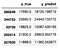Compare of actual and predicted values

From the prediction, we can see that its accuracy is 68.4% with the average error of 5105.99 degrees. The table compare the result of actual y and the predicted y

# Conclusion

With this, we cover lots of things from visualizing data, cleaning data, evaluating models, and do the prediction. The accuracy of the model might not be so high but we get to see the process of implement the regression prediction. To improve the prediction or model training, we can apply the adjustment in the model hyper parameter or select only most relevant attributes for our model.

## Analytics Vidhya

Analytics Vidhya is a community of Analytics and Data…

## Sign up for Analytics Vidhya News Bytes

### By Analytics Vidhya

Latest news from Analytics Vidhya on our Hackathons and some of our best articles! Take a look.

By signing up, you will create a Medium account if you don’t already have one. Review our Privacy Policy for more information about our privacy practices.

Check your inbox
Medium sent you an email at to complete your subscription.

## Analytics Vidhya

Analytics Vidhya is a community of Analytics and Data Science professionals. We are building the next-gen data science ecosystem https://www.analyticsvidhya.com

Written by

## Meimi Li

A graduate from business intelligence major who fascinate about Machines learning and Artificial Intelligence.## Analytics Vidhya

Analytics Vidhya is a community of Analytics and Data Science professionals. We are building the next-gen data science ecosystem https://www.analyticsvidhya.com

## More From Medium

Medium is an open platform where 170 million readers come to find insightful and dynamic thinking. Here, expert and undiscovered voices alike dive into the heart of any topic and bring new ideas to the surface. Learn more

Follow the writers, publications, and topics that matter to you, and you’ll see them on your homepage and in your inbox. Explore

If you have a story to tell, knowledge to share, or a perspective to offer — welcome home. It’s easy and free to post your thinking on any topic. Write on Medium